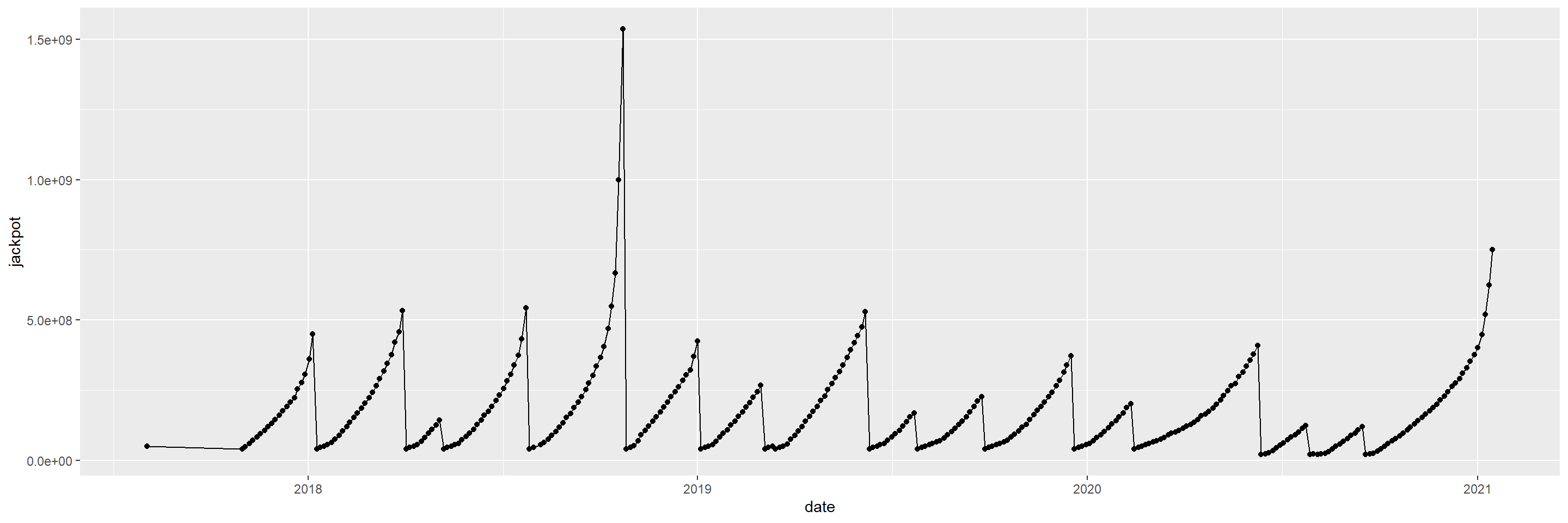# Overview

The goal in this analysis to:

• examine basic jackpot and sales data for the Mega Million lottery since October 2017 (when current rules went into effect)
• calculate the likelihood of there being a split jackpot, depending on how many people bought tickets
• visualize how the annuity value and cash value of the jackpot have changed over time
• calculate how much winnings are reduced by federal and state (CA) taxes
• calculate the expected value of every Mega Millions tickets since October 2017, after adjusting for:
• cash value of ticket
• taxes
• likelihood of splitting the pot

# — Settings —

``````knitr::opts_chunk\$set(echo = TRUE)
plot_save <- FALSE``````

# — Packages —

``````library(readxl)
library(tidyverse)
library(lubridate)
library(binom)``````

# — Load Data —

## 1. Read

``lott <- read_xlsx("lottery_sales_data.xlsx")``

## 2. View

``View(lott)``

## 3. Types

``````# examine cols&types
t(data.frame(lapply(lott, class)))``````
``````##          [,1]      [,2]
## date     "POSIXct" "POSIXt"
## sales_mm "numeric" "numeric"
## sales_jj "numeric" "numeric"
## jackpot  "numeric" "numeric"``````

# 1. Basic Shaping & Viz

The goal here is to just get the data into a nice, workable format. And to do some quick and dirty, cursory visualizations, to get a sense of what the main variables of interest (jackpot sizes, and total sales) look like.

## 1.1. Shape

``````lott_clean <-
lott %>%
mutate(jackpot = jackpot * 1000000,
sales = sales_mm + sales_jj)

lott_clean``````

## 1.2. Graph: Just Jackpot

Graph of jackpot sizes over time. Axes and everything still ugly.

``````ggplot(data = lott_clean,
aes(x = date,
y = jackpot)) +
geom_point() +
geom_line()``````## 1.2. Graph: Just Jackpot (NICE)

``````color_jackpot <- "green4"

plot_just_sales <-
lott_clean %>%
mutate(jackpot = jackpot / 1e6,
sales = sales / 1e6) %>%
ggplot(aes(x = as.Date(date))) +
geom_line(aes(y = jackpot),
color = color_jackpot,
size = 2,
alpha = 0.5) +
scale_x_date(name = "Date",
date_labels = "%Y",
date_breaks = "1 year") +
scale_y_continuous(labels = c("\$0", paste0("\$", seq(1, 9, 1)*100, "m"), paste0("\$", seq(1, 1.6, 0.1), "b")),
breaks = c(0, 1:16*100),
name = "Jackpot Value \n (m=million, b=billion)") +
labs(title = "Jackpot Size Over Time") +
theme_bw() +
theme(plot.title = element_text(hjust = 0.5,
size = 15),
axis.text.y.left = element_text(color = color_jackpot,
size = 15),
axis.title.y.left = element_text(color = color_jackpot,
size = 15,
margin = margin(t = 0, r = 10, b = 0, l = 0)),
axis.text.x = element_text(angle = 0)
)
plot_just_sales``````